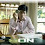## Thursday, August 9, 2012

### OP AMP - part 1

The OPAMP related objective questions are given in this post with answers.

1. A non inverting closed loop op amp circuit generally has a gain factor

A. Less than one
B. Greater than one
C. Of zero
D. Equal to one

Ans: B. For non inverting amplifier the gain is A = [1 + (Rf/Rin)]. So it will be always more than one
2. If ground is applied to the (+) terminal of an inverting op-amp, the (–) terminal will
A. Not need an input resistor
B. Be virtual ground
C. Have high reverse current
D. Not invert the signal

Ans: B. Other options are not suitable.
3. The closed-loop voltage gain of an inverting amplifier equal to
A. The ratio of the input resistance to feedback resistance
B. The open-loop voltage gain
C. The feedback resistance divided by the input resistance
D. The input resistance

Ans: C
4. When a number of stages are connected in parallel, the overall gain is the product of the individual stage gains
A. True
B. False

Ans: B
5. An ideal OP-AMP is an ideal
a) Current controlled Current source
b) Current controlled voltage source
c) Voltage controlled voltage source
d) voltage controlled current source

Ans: C. The ideal Opamp output voltage is maintained constant. It is controlled by input voltage.
6. The ideal OP-AMP has the following characteristics.
a) Ri=∞ ,A=∞ ,R0=0
b) Ri=0 ,A=∞ ,R0=0
c) Ri=∞ ,A=∞ ,R0=∞
d) Ri=0 ,A=∞ ,R0=∞

Ans: A.
7.Calculate the cutoff frequency of a first-order low-pass filter for R1 = 2.5kΩ  and C1 = 0.05μF
A. 1.273kHz
B. 12.73kHz
C. 127.3 kHz
D. 127.3 Hz

Ans: A.

Hint: low pass filter cut off frequency f = 1/(2πRC)
8. How many op-amps are required to implement this equationA. 2
B. 3
C. 4
D. 1

Ans:D. The output voltage of inverting amplifier is Vout = (-Rf/Rin)Vin. By keeping 1 inverting amplifier and three no of sources (V1, V2, V3) in series corresponding input resistors(R1, R2, R3), we can get this equation.
9. How many op-amps are required to implement this equation Vo = V1
A. 4
B. 3
C. 2
D. 1

Ans: D. The voltage follower which has one opamp has the output of Vo = Vin
10. An OPAMP has a slew rate of 5 V/μ S .The largest sine wave O/P voltage possible at a frequency of 1 MHZ is
A.  10 volts
B.  5 volts
C.  5/ volts
D.  5/2 volts

Ans: D
Hint: Slew rate is defined as the max. rate of change of output voltage. Its unit is V/μS.
Time period = 1/f = 1/1MHz = 1μS
V= Vm. sin(
ωt) = Vm. sin(2πf.t)

slew rate = dV/dt = d(Vm. sin(2πf.t)/ dt=Vm. 2πf. cost...

5:56 AM

1.i want the solutions also..kindly look over it

2.1.3.4.5.Question Number 5: OPAMP is a Voltage Controlled Voltage Source

Question Number 8: The output equation is the output for an inverting Summing Amplifier. So this expression can be implemented using a single OPAMP

6.Question Number 5: OPAMP is a Voltage Controlled Voltage source

Question Number 8: The given equation is the output of an Inverting Summing Amplifier. So we can represent it using a single OPAMP

1.Thanks ami... we regret for the mistake and corrected them.

7.hey you my friend here some answers are wrong
8)1 op amp can required for that eqn

1.Thanks for your suggestion. We regret for the mistake. We corrected it.

8.9.